# Paul McWhorter arduino lesson 12

Those are the pins 1 through 13. These are pins that we write voltages to. We remember that the the pins that don’t have a squiggly line. We can do digital rights to that. Would be a digital right to that pin high or to that pin low, but if we use the pins that have the squiggly line by them, we can actually write an analog voltage and we write an analog voltage by writing a value between 0 and 255. If we write the value 0 to one of those squiggly line pins, we write a 0 volts. If we write a 255, we write 5 volts, so we can write voltages between 0 to 255 on pins, 3, 5, 6, 9, 10 and 11. Along that, along that bottom row, the other pins were able to just write only able to write digital values to higher low, ok, but so far all we’ve been doing with our circuit. Well, we’ve been talking to the user over the serial port printing messages to him over the serial port and then getting input from him over the serial port. So we can interact with the user and then we can make things happen to the circuit. We can apply voltages to the circuit, we can apply digital voltages or we can apply analog voltages. So those are the things that we’ve learned so far. Now, what we’re going to do today is we’re not just going to be talking to the circuit we’re, going to be listening back from the circuit we’re going to be going out in making reads, we’re going to be reading voltages off the circuit and to see how To do this, we want to start with, like the simplest simplest example possible.

So basically, what we have here is a potentiometer if you’re not familiar with how a potentiometer works, go back and work, video go back and watch video number 9 and video number 9. We go through some of the circuit diagrams for how to how to work with a with a potentiometer and how to make a simple voltage divider being. This is just a quick schematic of the voltage divider that we’re trying to achieve the voltage in it’s. Basically, just a fixed voltage, it’s five volts the way in the circuit that we’re getting the the voltage in is we’re coming off and we’re getting 5 volts off of the Arduino from the 5 volt pen coming down and just establishing a 5 volt rail on our On our breadboard and then we bring that 5 volts and we go to the top leg of the potentiometer. So the 5 volts comes then goes to the pop top leg of the potentiometer, where we’re going to read, is and we’re going to read the voltage off. The center leg of the potentiometer, so you can see this – is the center pin on the potentiometer we’re, going to bring that up to the analog pin 0, which is right here so by coming from the center pin of the potentiometer up to a 0 it’s. Going to be that a 0 pin that we use to read a voltage off of the potentiometer and then down on the lower leg of the potentiometer, we bring that over to our ground, rail and that ground rail is our top row.

And so we bring that. Third leg up to the top row and then that top row we connect to ground on the Arduino, and so basically, what we create is a very simple voltage, divider that’s shown here and what the resistance this RP is. The resistance of the potentiometer let’s say for this it’s, a thousand ohms. Well, basically, that thousand ohms is split between r1 and r2, depending on where you have the knob set. If you have the knob set all the way to the left, all tidge appears as as r1. There is no r2 and therefore the voltage out is going to be zero it’s going to be like you’re looking at the ground. If you turn it all the way to the right for this particular particular potentiometer, it puts all the resistances R. There is no r1 V out seized the end, so it would see 5 volts. So, basically, with this circuit, our fee out is the center leg of the potentiometer. Just like you see here the center leg of the potentiometer and by hooking this circuit up like this, what you should see happen is, if you read a voltage on, pin a zero. You should be able to vary that voltage between zero all the way up to five volts by turning this little knob. Okay, if I turn it to the left, I should be getting zero volts. You know if I turn it all the way to the right.

I should be getting five volts. Okay, now, one of the things to understand when we do an analogue read it’s kind of like remember when we did the analog right, that things were scaled and so, if we wanted to write zero volts, we would write zero. We would write a value of zero if we wanted to write five volts. We would write a number of 255 to that pen, so a right value of 255 corresponding to a voltage of 5 volts, and so we had to scale things well. When we do analog readings, we have to scale things as well. Although the scale factor is a little bit different, the analog numbers, the numbers that we will read off of that analog pin, will be between 0 and 10 23. Okay, if we connect and try to read 5 volts, the read value that we will get will be 10 23. If we read zero volts, the revalue that we will get will Z will be 0. So, basically for voltages between 0 amp 5, we will get read values between 0 and 10, 23 and so you’ve got a scale that number that you read that’s between 0 to 1023 you’ve got a map it on the number 0 to 5 and we’ll go through The math on that in a minute, but for right now, let’s just see if we can make of a measurement on this voltage divider on this potentiometer and let’s, see if we can get values that are what we’re expecting let’s see.

If we can make this thing work, so in order to do that, what we need to do is we need to call up a nice new, Arduino and integrated development window, and we will start writing some code here. Well, if we’re going to talk to, pin Z a zero, if we’re going to work with, pin a zero, we probably should assign a variable to it so that we can go through and write our program based on using that variable. And so what we can do is we can set it up as an int and then what am I going to call it well it’s hooked to my potentiometer so I’m going to call it my pot pen, all right and that’s, going to be equal to a Zero, you try to display a zero all right now, the thing to understand is normally anytime. You do an integer declaration. You would just put a number in here but it’s unique, that it will recognize as integers those pin names and we are connected to an a zero this. We are ok and then what we are doing is we’re assigning a SS assigning a pot pin to a zero. We try to be good and put our. We try to be good and put our our comments in here now we’re going to we’re going to be reading from that 10, so we’re going to need a variable to put that number into. So we need to declare a variable it’s going to be an integer and we are going to declare it we’re going to call that our read value, so the value that we read from 10 8 0 we’re going to call that read value we’re, declaring the variable Here but we’re not assigning a value to it, because we’ll get the value from the pin.

We’Ll go out and we’ll read it from the pin. So we’re not assigning it here, but we are declaring our read value. Variable. Ok, now, let’s do our pin modes. Let’S come in our void setup, which pen are we going to be doing? We are going to be working with our pot. Pin, okay, that’s pin a 0, but what is it going to be always up until now, our pins have been output because we’ve been outputting to the pins. This pin we’re going to read from so this is going to be an input, so we’re going to say 10 mode, and then it is the pin pot pot pen and it is going to be an input. I think this is the first time we played an input variable also. We are going to be working over the serial port, interacting with the user, so we need to turn the serial port on so we’ll. Do our serial dot, B again and I’m, going to go to a 9600 baud this time right just make sure that whatever baud you use, it matches the bod. That said in the serial in the serial port and the serial monitor, okay, look I’ve declared my variables I’ve done my pin mode and I’ve started my serial port. So what can we do down here? Well, let’s see if we can make a reading off of that. Pin so the way to make an analog reading is you do analog, read notice the bumpy font and the R is uppercase, so analog read with our uppercase, you see it turned orange.

As I slowly turn it to the right I’m getting more and more and more resistance coming from up here down to here, so this is like not pointing here it’s pointing further and further and further towards the top 526 I’m about halfway there because remember 0 to 5 volts will read 0 to 1023, and so a value of 526 527 corresponds to about 2 and a half volts so I’m about halfway to turning it all the way to the right and so I’ll continue to turn it here. You can scroll in growing growing and then I turned it all the way to the right, and I get it all the way up to 10 23 when it’s reading 10 23 that corresponds to a voltage of 5 volts. You know everything scales from 0 to 1023 represents numbers from 0 to 5, volts, ok, so now what you can see here is is that I have a little input device and the number that I get on my serial monitor depends on where I set this potentiometer. Like I have a little knob like that, could be a volume control now I could set the brightness of the LED, based on where I put this or I could set other things based on, where I put that so now I don’t have to just get user From the input over the serial monitor, I could set things based on where I put this potentiometer. So now all the sudden it’s like a little product where I could start turning that knob and getting the LED brighter or dimmer.

So we have one point: the point: zero, zero, alright what’s. The second point we know. The second point we know up here is that a read value of 10 23 corresponds to a world voltage of 5. So we have a second point of 10235. So we have two points for this line. If we have two points, we can calculate a slope. Slope is y2 minus y1 over x2 minus x1 that’s going to be equal to let’s. Have this, as as the second point in this is the first point, makes it a little easier. I like to do my linear equations to try to avoid negative numbers, so I have 5 minus 0 over 10, 23 minus 0, so that’s Y 2, minus y1, y2. Minus y1 over x2 minus x1 and that comes out to be 5 over 10 23. Now we know the slope. The point: slope form of a line is y. Minus y1 is equal to M over m times X. Minus x1. Your point: x1. Y1. You could pick this point where you picked that point and you’ll get the same answer, but it’s a lot easier. If you pick the easier point, let’s pick this one all right now, let’s put in the values Y minus y1 is M onto X, minus X, 1 Y minus 0 right. Ok, that’s 0 is equal to 5 over 10 23, which was the slope times X. Minus x1 all right times X, minus next one, so we have 5.

Minus 0 is equal to 5 over 10 23, the slope on 2 X, 0 and that’s, because we chose this point of zero zero to make it easier. The other one would have eventually given us the same answer. So Y is equal to 5 over 10 23 times X. That is the equation of this line. Now the only thing I’m going to do now, I should switch it to our y axis Y was voltage and X was read value, so we change Y for voltage and X. We make read value, so what we see is the real world voltage is equal to 5 over 10 23 times the read value. Okay – and these are the read values that we’re getting here, and so we can use this equation that we got from using that equation of a line that you had to learn place in seventh grade and wondered when you were ever going to use it well, you’re Using it now to allow you to really create an equation that will take this read value. This read number that we’re getting that’s between 0 to 1023 and turn it into a real world voltage a real voltage that’s between 0 and 5. So let’s go ahead and see if we can do that, so we’ve got our revalue. Let’S create another variable called integer and it’s our voltage – and this is our real voltage we’re going to calculate this. So we are not going to assign a value to it.

We’Re just going to declare it ok, declare our volt voltage variable okay, now notice how didn’t it just out a habit, but I want you to think about this. Are we interested in just the voltage? 1. 2. 3. 4. 5. There could we have in between voltages? Well very much we could have in between voltages and we could have a whole lot of in between voltages. We could have 0.1 volts point to point 3. Point 4. We could have very, very, very small, increments in voltage, and so really we want the in between numbers. So we should not declare this an int. We did should declare it a float and again when we declare something as float. That means a floating point number. If we go back to math class, that would be equivalent to a real number it’s all in between numbers, and we call them floats in programming language. So we have a float variable now we’re going to calculate what is our real world voltage? Will the voltage the voltage is going to be equal to remember it’s, going to be 5 over 10 23, but now this is very, very, very important and I mean over and over. I see students forget to do this if we are declaring something to be a float and if we are using floating numbers, we have to write our numbers as floating numbers and sit so instead of saying it’s 5 over 10 23 that’s 5 over 10 23.

When our dueo sees 5, it sees it as an int and when it sees 10 23, it sees it as an int. And so, if you do, the int math integer math of 5, divided by 10 23 it’s, going to come back with a 0 because it’s going to round it to 0 or it’s, going to truncate it to 0. And so you don’t want it. Because you want a real floating point, number well, we’ve said voltages floating points, so we need to make these floating point numbers and we do that by putting a decimal. Five point tells Arduino that you want the floating point: number 5, not the integer 5 and similarly here point so when you are doing floating point math on the Arduino, make sure that you put your decimal in or you’re going to get some surprising results. That’S going to take a long time to figure it out, so what was it? Voltage is equal to 5 over 10 23 times Reed value, and so we have 5 as a float divided by 10 23 as a float and then we’re going to go times. Reed value, okay and then we’re going to do this is what is it doing? It’S calculating the real world voltage? Okay, now let’s download this and see if it’s still happy looks like it’s going to be happy see if we can get our serial monitor back up. Call that thing up all right now.

So you see why we needed floats, because we need the numbers between the integers and so now I can turn it up and I can get 0.5 volts let’s like let’s see I can get about I’m trying to get about 175 it’s kind of hard to get It exactly about 7 5. I can take it up to one volt. Take it on up, keep turning to the right, keep turning turning keep turning, I get five volts. Yes, you see what I’m doing is I’ve got this potentiometer. Okay, I did the math and it was based on this voltage divider that we talked about okay let’s, see if we can get it all, showing here and I’m reading. Now an analog voltage off of that voltage, divider and I’m reading it in real volts from zero to five volts, so I’m reading, an Arduino number that’s between zero and ten twenty three and I’m, converting that to a real world voltage between zero and five volts in Cc it’s like a little volt meter that we would that we built here and the voltage corresponds to whatever voltage that this, that this pin, that this thing analog analog, pin reads: well, let’s just do an experiment! Okay, what? If I just unplug this from the potentiometer? Okay – and I put it on my ground, what should it read if I put on the ground, it should read zero volts. I didn’t put it on the ground.

We’Ll, do digital writes the ones with the squiggly lines will do analog, writes and the ones that do not have the squiggly lines will just do digital rights and so squiggly line on the on this bottom set of pins will do analog, writes or digital writes the Ones without the squiggly lines will do only digital rights. Okay, so this is a powerful tool, a new tool we have now we can talk to the user over the serial monitor. We can read values from the serial monitor. We can apply voltages. Digital voltage depends. We can apply analog voltages to pins, and now we can read analog voltages, so our toolset is getting pretty pretty sophisticated and we’re going to be able to do some pretty impressive things. With these simple tools fairly quickly. Okay, again, I am Palmer quarter.

# Paul McWhorter arduino lesson 12 Social

(Visited 49 times, 1 visits today)

## Comment (34)

1.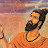Vinay Verma says:

loved the explanation!!!THE BEST TUTORIAL FOR SURE

2.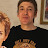Rod Henderson says:

Hi Paul 😀 great vids- i’m learning more from this than i did at TAFE lol- my formula is a little different i used this code:
Serial.println(j);
delay(1000);

3.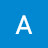Any Bag says:

The P.O.T. my Arduino kit came with is a large one with a knob will that make a difference?

4.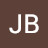JB deCABOURG says:

You make a mysteque 0 to 1023= 1024 so you need to do 5/1024 and not 5/1023

5.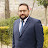aqib shafiq says:

hy.any body guide me about tutorial to read analog value of pulse senor thorough arduino and bluetooth hc 05 and send it to laptop

6.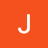Joseph Jorgensen says:

Why dose a analog read 1023 be five volts but analog write is 255 why not just 255 again

1.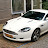mdsabir shaik says:

Because reading analog (A0,A1…A5) send info to processing units using 10 bit pipeline in decimals =2^10 =1024

And writting (pin 1,2,…13) using pipeline 8 bit in decimals = 2^8 = 256

One is less in both because we also count zero …

2.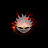ADmagma says:

@mdsabir shaik thanks I had that question too

7.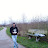TechnoVan says:

hi sir i appreciated your Videos but i think most of them , you are not explain about Arduino itself but about maths, that it is not what we are looking for , what we are looking for is programming , Codes what they do, math is boring

1.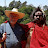Paul McWhorter says:

Actually the math is very important. If you do not understand the math, you will never be a successful engineer or scientist. My goal is to train people to become successful, not create ignorant coders.

2.TechnoVan says:

@Paul McWhorter Sir with all do respect when you go home and push the button to turn the lights ON or OFF in your house, you don’t care what AC volts are 110v or 220v behind the button ,what is really matter if the lights is ON or OFF, when you want to take things 1 meter far way from you i don’t need to know that 1 meter has 100 centimeters , i just want 1 meter , simplify thing it is part of life make life easy! sorry if i bother with my ignorance, just because you knowhow 2 + 2 = 4, it does not make you a genius, have a awesome day sir

3.Paul McWhorter says:

I think you just dont get it . . . when you turn the light switch on, you want more to happen than you are saying. When you turn the light switch on, you want the light to turn on, and you want the house to NOT burn down. If you do not do the math, you have not calculated the current carrying capacity of the wire you put in the wall, the correct breaker to use, and how many outlets can be put on the circuit. You do this job without the math, and maybe the light comes on and the house burns down. Luckily, successful, practicing engineers and electricians have better judgment than your comment reflects.

8.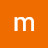mcsniper77 says:

I get the concept of commenting. But with this language you can make commands so obvious that there is little point. Like come on it’s not G-code.

9.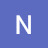Ned Noah says:

for the math i just did 1023/5= 204.6 n so readValue/204.6 = voltage. i dunno about all this line and graph stuff haha i mean like it seems unnecessary haha

1.Paul McWhorter says:

You have a light sensor that reads 129 in the dark and 1264 when the lights are full bright. you want to output a voltage between 2.5 and 5 volts depending on brightness between dark and full bright. You want output voltage to change smoothly between the two values based on brightness.

2.Ned Noah says:

@Paul McWhorter Thank you sir

3.Ned Noah says:

@Paul McWhorter I know the math is still the same, but, just wondering, wouldn’t the maximum value for the light sensor be 1023? I mean at full brightness

4.Paul McWhorter says:

No, the sensor changes resistance based on how bright the light is . . .ends up between two values you have to measure. Might never get to 1023.

5.Ned Noah says:

@Paul McWhorter Ahhh yes, sorry, you said sensor reading and I was thinking about the maximum value for the potentiometer reading being 1023.. I see. Thank you for responding. Much appreciated

10.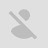sublexxus79 says:

But why not int potPin and int potValue ?

1.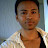boowonder888 says:

Indeed, potValue would have been the clearer name.

11.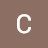Captain Prototype says:

Can you expect the same values if you use a PWM pin instead of the analog pin?

1.Captain Prototype says:

The PWM can only do digitalWrites and analogWrites. PWM does not do any Reads, period!

12.Captain Prototype says:

In fact, can you use a pwm pin with a pot?

13.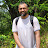spectrum1844 says:

Great Video – this makes me think – how can this circuit be modified to read lets say up to 50 Volts ? need some chip that takes 5 volts and can output max 50v?

1.Paul McWhorter says:

You really need to know what you are doing as you use higher voltages. With simple low current 5 volt inputs and outputs things are safe, and nobody gets hurt. As you deal with higher voltages and currents, make sure you know what you are doing.

14.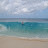moustick2025 says:

very well explain, keep good work

15.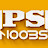Sree Ram says:

Tnks

16.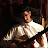Muhammad Hammad Umer says:

Sir ur lessons are Awesom…I have aQuestion related to this
Q1: I have used 3.3V pin instead of 5 volt pin but Serial Monitor shows output voltage upto 3.5V , 3.6 V , 3.7 V , 3.8V why is it so ?

17.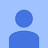2ftpmarco says:

very very good way of teaching !

18.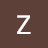Zeeshan Faraz says:

i can’t get this to print Strings along the Voltage Float values

EDIT : My bad, it was the bloody cable not letting me do it.

19.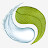Erygion says:

Great lesson Mr. Mcwhorter. I’ve been down this road before but have since gotten rusty…I took notes and you make it clear and concise. Thank you.

20.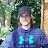Louis Ferron says:

Could someone help me with this problem, thanks!
I tried out the sketch from this video with the same setup and everything worked except for the serial monitor; it gives me values between 0 and 1023 but it updates very slowly. For example, if I turn the potentiometer from one end to the other, the read values have a delay of 250ms but the values given are approximatly 30sec late than what is physically happening with the potentiometer. So I don’t really know what problem could be

1.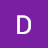Dax Hopkins says:

Is it an official arduino? If not, which brand?